Next: Isospin mixing Up: Isospin mixing in nuclei Previous: Introduction

# Theory

The isospin-projected DFT technique [11,12,13] utilizes the ability of the self-consistent MF method to properly describe the balance between the long-range Coulomb force and the short-range nuclear interaction, represented in this work by the Skyrme-type energy density functional (EDF). To remove the spurious isospin-symmetry-breaking effects, we use the standard one-dimensional isospin projection after variation, which allows us to decompose the Slater determinant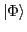into good isospin states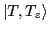:(1)

Here,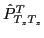stands for the conventional one-dimensional isospin-projection operator: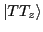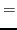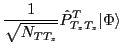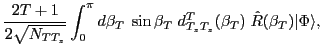(2)

where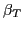denotes the Euler angle associated with the rotation operator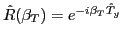about the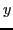-axis in the isospace,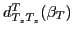is the Wigner function , and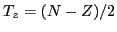is the third component of the total isospin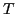. The normalization factors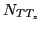, or interchangeably the expansion coefficients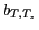that encode the isospin content of, read: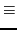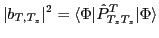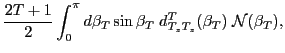(3)

where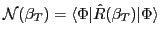is the so-called overlap kernel. For technical aspects concerning the calculation of the overlap and Hamiltonian kernels, we refer the reader to Ref. . The isospin-projected DFT technique utilizes the ability of the HF solver HFODD  to produce fully symmetry-unrestricted Slater determinants.

The isospin projection determines the set of good isospin states (called the basis in the following), which in the next step is used to rediagonalize the entire nuclear Hamiltonian, consisting of the kinetic energy, Skyrme EDF, and the isospin-breaking Coulomb force. The rediagonalization leads to the eigenstates: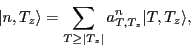(4)

numbered by index. The amplitudes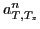define the degree of isospin mixing through the so-called isospin-mixing coefficients (or isospin impurities) for the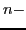th eigenstate: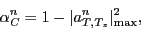(5)

where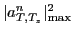stands for the squared norm of the dominant amplitude in the wave function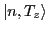. It is worth stressing that the isospin projection, unlike particle-number or angular-momentum projections, is essentially non-singular; hence, it can be safely used with the local EDFs. The rigorous analytical proof of this useful property can be found in Ref. .

The combined isospin and angular-momentum projection leads to the set of states,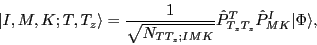(6)

which form another normalized basis built on. Here,and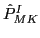stand for the isospin and angular-momentum projection operators, respectively, and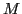and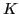denote the angular-momentum components along the laboratory and intrinsic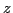-axes, respectively . Now the problem becomes more complicated because of the overcompleteness of the basis (6) related to the-mixing. This is overcome by performing the rediagonalization of the Hamiltonian in the so-called collective space, spanned for each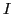andby the natural states,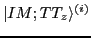, as described in Refs. [18,20]. Such a rediagonalization gives the solutions: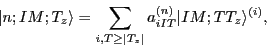(7)

which are labeled by the indexand by the conserved quantum numbers,, and[cf. Eq. (4)].Next: Isospin mixing Up: Isospin mixing in nuclei Previous: Introduction
Jacek Dobaczewski 2011-02-20